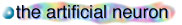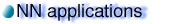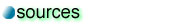The perceptron

The perceptron is a mathematical model of a biological neuron. While in actual neurons the dendrite receives electrical signals from the axons of other neurons, in the perceptron these electrical signals are represented as numerical values. At the synapses between the dendrite and axons, electrical signals are modulated in various amounts. This is also modeled in the perceptron by multiplying each input value by a value called the weight. An actual neuron fires an output signal only when the total strength of the input signals exceed a certain threshold. We model this phenomenon in a perceptron by calculating the weighted sum of the inputs to represent the total strength of the input signals, and applying a step function on the sum to determine its output. As in biological neural networks, this output is fed to other perceptrons.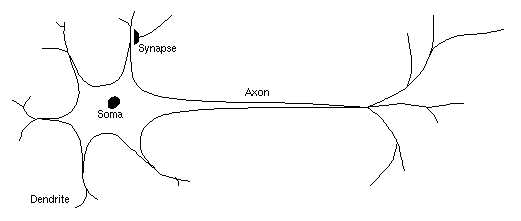(Fig. 1) A biological neuron(Fig. 2) An artificial neuron (perceptron)

There are a number of terminology commonly used for describing neural networks. They are listed in the table below:
 The input vector All the input values of each perceptron are collectively called the input vector of that perceptron. The weight vector Similarly, all the weight values of each perceptron are collectively called the weight vector of that perceptron.

What can a perceptron do?

As mentioned above, a perceptron calculates the weighted sum of the input values. For simplicity, let us assume that there are two input values, x and y for a certain perceptron P. Let the weights for x and y be A and B for respectively, the weighted sum could be represented as: A x + B y.

Since the perceptron outputs an non-zero value only when the weighted sum exceeds a certain threshold C, one can write down the output of this perceptron as follows:

 Output of P = {1 if A x + B y > C {0 if A x + B y < = C

Recall that A x + B y > C and A x + B y < C are the two regions on the xy plane separated by the line A x + B y + C = 0. If we consider the input (x, y) as a point on a plane, then the perceptron actually tells us which region on the plane to which this point belongs. Such regions, since they are separated by a single line, are called linearly separable regions.

This result is useful because it turns out that some logic functions such as the boolean AND, OR and NOT operators are linearly separable ş i.e. they can be performed using a single perceprton. We can illustrate (for the 2D case) why they are linearly separable by plotting each of them on a graph: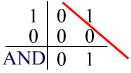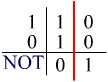(Fig. 3) Graphs showing linearly separable logic functions

In the above graphs, the two axes are the inputs which can take the value of either 0 or 1, and the numbers on the graph are the expected output for a particular input. Using an appropriate weight vector for each case, a single perceptron can perform all of these functions.

However, not all logic operators are linearly separable. For instance, the XOR operator is not linearly separable and cannot be achieved by a single perceptron. Yet this problem could be overcome by using more than one perceptron arranged in feed-forward networks.(Fig. 4) Since it is impossible to draw a line to divide the regions containing either 1 or 0, the XOR function is not linearly separable.